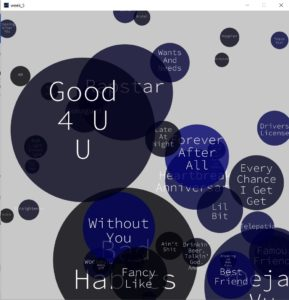# Week 4(Data Analysis)

#### Description:

I wrote a code that reads from the billboard top 100 songs (not the most recent). I edit the .csv file I found online to remove redundant data that I did not need and I used the left data to draw circles at random locations for each of the top 100.I added a small alpha value to show the layering of circles as they are random.

I had to deal with longer song names and make them wrap around.

Instead of trying to find a library that works, I decided to try to make the wrap work myself to explore and know what I can do to manipulate a string.

#### Code:

I started with a circle class to draw each circle at a random location and depending on circle details. I added an array to save the circles.

```String names[];
int ranks[];
int weeks[];
boolean arrDone[] = new boolean;
boolean flag = false;
int counterDone = 0;

PFont myFont;

class Circles {
//attributes
PVector pos ;
int col;
int diam;

String text;

// number of weeks on board gives color shade, and rank determines radius
Circles(int weeks, int ranks, String _text) {
pos = new PVector(random(0, width), random(0, height));
//calculate fraction from 255
col = int((255*weeks/87));
text = _text;
//max diam = 70
diam =int((width)/ranks);
diam = constrain(diam, 50, 500);
}

void draw_circle() {
fill(0, 0, col, 200);
//fill(255,100);
noStroke();
ellipse(pos.x, pos.y, diam, diam);

// split text into lines
String[] words = split(text, ' ' );

writetext(words);
}```

The next function is part of the circle class, I put it here separately because it includes the code for the wrap of the text.

```void writetext(String[] arr) {
String[] temp = new String[arr.length];
int n=0;
for (int i=0; i<arr.length; i++) {
fill(255);
textSize(diam/5);
textAlign(CENTER);
if (arr.length>1) {
if (i<arr.length-1) {
if ((textWidth(arr[i])+textWidth(arr[i+1]))<diam/2) {
temp[i] = arr[i] + " " + arr[i+1];
n++;
//i++;
continue;
} else {
temp[i] = arr[i];
n++;
}
} else {
temp[i] = arr[i];
n++;
}
} else {
temp[i] = arr[i];
n++;
}
}
for (int k=-1; k<n-1; k++) {
if (arr.length>1 && textWidth(temp[k+1])>0) {
if (n>=3) {
text(temp[k+1], pos.x, pos.y+k*diam/5);
} else {
text(temp[k+1], pos.x, pos.y+(k+1)*diam/5);
}
} else {
text(arr, pos.x, pos.y+(k+1)*diam/5);
}
}
}
}```

Here, I declared the circle’s object array and then loaded and processed the data from the .csv file.

```void readData() {
String data[]= new String;
// Convert String into an array of integers using ',' as a delimiter
// string array is returned, which we cast to an int array
names = new String[stuff.length];
ranks = new int[stuff.length];
weeks = new int[stuff.length];

for (int i=1; i<stuff.length; i++) {
data = split(stuff[i], ',' );

//fill arrays with data
ranks[i] = int(data);
names[i] = data;
weeks[i] = int(data);
}
}```

in the creat function here I added a boolean array to check if the song has been output on the screen or not to avoid repitition.

```void create() {
int num =  int(random(1, 99));
boolean flag = false;
if (arrDone[num]) {
flag= true;
} else {
arrDone[num] = true;
}

if (!flag && count<=100) {
// int weeks, int ranks, String _text){
circle[count] = new Circles(weeks[num], ranks[num], names[num]);
circle[count].draw_circle();
count++;
}
}
```

I only used the draw function for the text to appear at the beginning of the code running time. I generated the circles with mouse clicks instead to give time for the user to analyze each new circle.

```void setup() {
size(1000, 1000);
background(200);
}

void draw() {
myFont = createFont("SourceCodePro-Light.ttf", 60);
textFont(myFont);
if (frameCount<25) {
String textt = "Press at a random location";
textSize(60);

text(textt, width/2-(textWidth(textt)/2), height/2-32/2);
}else if(frameCount<30){
background(200);
}

}

void mouseClicked() {
if (frameCount>30){
create();
}
}```

## One thought on “Week 4(Data Analysis)”

1.Aaron says:

Looks great Fatema. Nice job on figuring out the text wrap. Are the sizes related to the songs’ positions on the top 100?